﻿ Blast Design Tools for Professionals | BlasToolsPro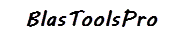# Features more than 100 formulas used in blasting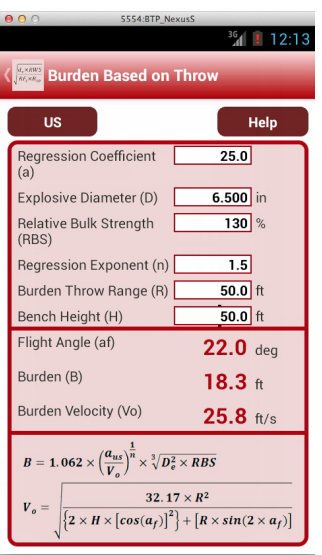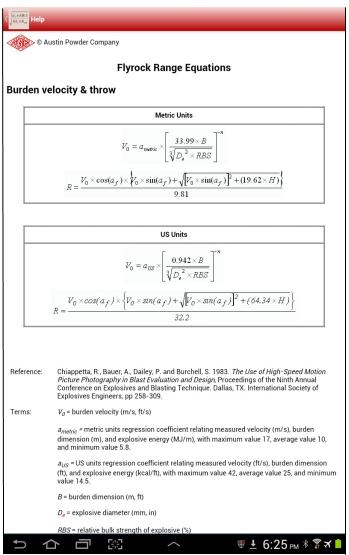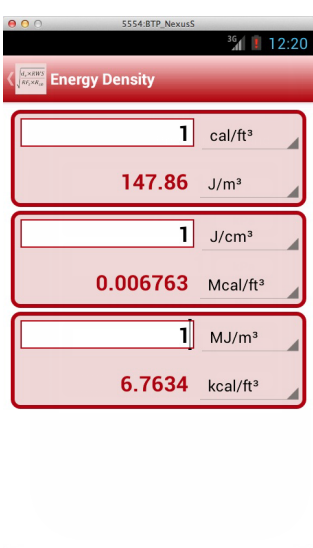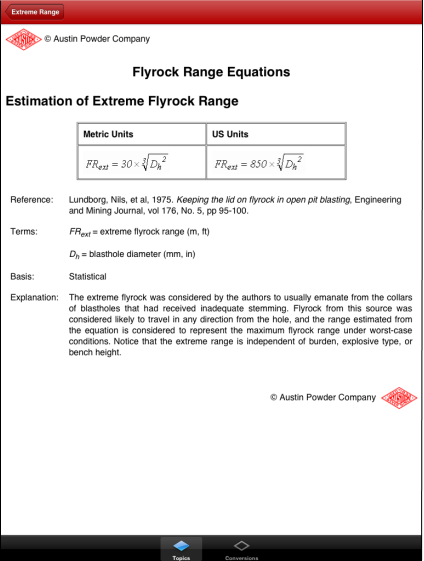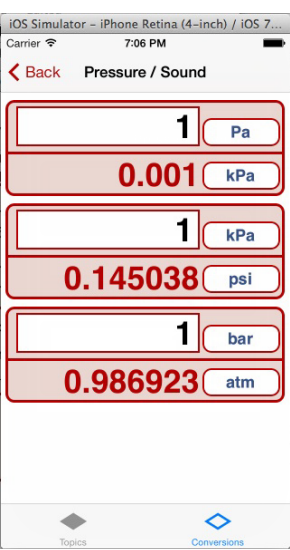BLAST PATTERN DESIGN
• Bench Height
• Hole Diameter
• Burden
• Spacing
• Sub-Drill Depth
• Top Stemming
• Deck Stemming
• Base Charge Length
DELAY TIMING
• Hole-to-Hole delays
• Row-to-Row delays
• Avoid supersonic face velocity
• Estimating vibration frequency
• Delay scatter
• Out-of-sequence probability
• Probability of close firing
VOLUME WEIGHT
• Volume of Rock per blast hole
• Weight of Rock per blast-hole
• Weight of Explosives per blast-hole
• Powder Factor
ANGLED HOLES
• Hole 'Look-out' distance
• Required hole angle
• Required hole length
• Burden - two angled holes
• Stemming - two angled holes
• Collar distance - two angled holes
PRE-SPLIT
• Pre-split spacing
• Pre-split stemming height
• Pre-split hole diameter
• Pre-split explosive diameter
DECOUPLED CHARGES
• Coupling Ratio
• Dropped explosive cartridge diameter
• Decoupled Charge Effective Density
WET HOLES
• No. of Cartridges to clear Water
• Final Height of Water in Wet Hole
ABNEY LEVELS
• Calculate Bench Height
• Calculate Toe Burden
• Face Angle
PRESSURE
• Detonation Pressure
• Blasthole Pressure
• Overpressure Prediction
VIBRATION
• Actual Scaled Distance
• Max Charge Weight
• Minimum Distance
• Predicting Vibration
• Site specific k factor
• Site specific b exponent
• Distance from seismic data
• Acceleration from PPV
• Displacement from PPV
• Explosive Quantity from PPV
• K Factor Converting
• Estimated p-wave velocity
FLYROCK
• Average Muckpile Displacement
• Extreme Flyrock
• Burden Velocity and Throw
• Burden Based on Throw
• Scaled Burial Depth
• Stem Length and Collar Flyrock Potential
FRAGMENTATION
• Rock Factor
• Uniformity Index
• Important Particle Size
• Convert D50% to Dxx%
STRENGTH
• Absolute Bulk Strength
• Absolute Weight Strength
• Relative Bulk Strength
• Relative Weight Strength
• Equivalent Weight of Explosive
GPS
• Latitude/Longitude to Distance
• Distance from UTM coordinates
• Distance from State plane coordinates
• Latitude/Longitude to UTM
• UTM to Latitude/Longitude
SUPPORTED CONVERSION TYPES
• Length - meters, kilometers, millimeters, centimeters, micrometers, thou, inches, feet, yard, miles
• Weight - kilograms, grams, milligrams, tonne, grain, ounces, pounds, ton, imperial ton
• Velocity - m/s, mm/s, km/s, in/s, ft/s, ft/min, ft/hr, mph, fu/fo
• Area - m2, mm2, cm2, km2, hectare, in2, ft2, yd2, acre, miles2
• Pressure/Sound - Pa, kPa, MPa, GPa, psi, kpsi, bar, kbar, atm, dB
• Volume - m3, mm3, cm3, liter, in3, ft3, yd3, gal
• Time - second, millisecond, minute, hour, day, week, month, year
• Density - kg/m3, t/m3, g/cm3, lb/ft3, lb/yd3, lb/gal, T/yd3, T/ft3
• Powder Factor - kg/m3, g/m3, lb/yd3, kg/t, g/t, lb/ton, t/g, t/kg, ton/lb
• Linear Charge Weight - g/m, mg/m, kg/m, grain/ft, lb/ft
• Energy Density - J/m3, J/cm3, kJ/m3, MJ/m3, cal/ft3, cal/cm3, kcal/ft3, kcal/cm3, Mcal/ft3, Mcal/cm3
• Specific Energy - J/kg, J/g, kJ/kg, MJ/kg, cal/g, cal/lb, kcal/lb, Mcal/lb, kcal/ton, Mcal/ton
• Power - watt, kilowatt, horsepower
• Temperature - Celsius, Fahrenheit, Kelvin